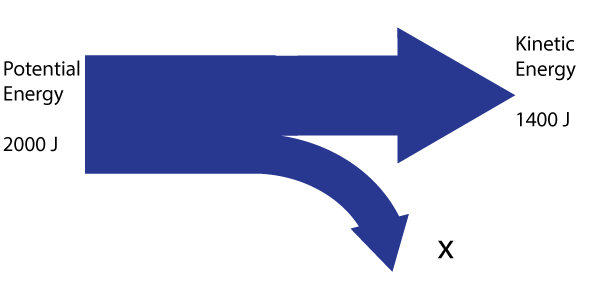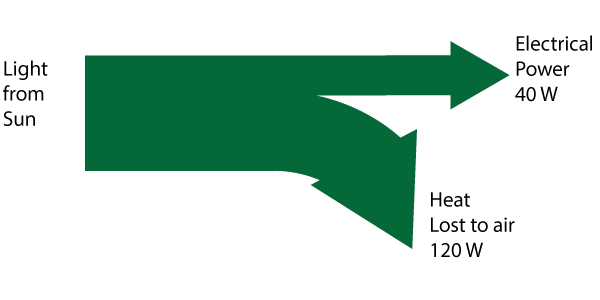µ GCSE | Efficiency

10 quick questions - for GCSE and iGCSE

10 minutes maximum! (can you do it in 5?)

1. Which of these is used to calculate the efficiency of an energy transfer or shift?

 A. useful energy output energy input B. energy input useful energy output C. energy input wasted energy output D. wasted energy output energy input

Q2-4. This diagram shows the energy transfer as a skateboarder rolls downhill:2. What is the percentage efficiency of this transfer of energy?

• A. 600 %
• B. 70%
• C. 30 %
• D. 1400 %

3. What form of energy is the wasted energy 'X' likely to be?

• A. Elastic energy stored in the skateboard.
• B. Chemical energy in the muscles.
• C. Wind energy from movement.
• D. Thermal energy from friction.

4. The energy in 'X' can be given as a value or as a percentage. Which of these is correct?

Energy in X (J) Energy in X (%)
A
600 30
B
1400 70
C
30 600
D
70 1400
5-7. This diagram shows the efiiciency of a solar panel:5. What does the 'W' represent in the measurment '40 W' in the diagram?

• A. Work Done
• B. Weight
• C. Wasted energy
• D. Watts

6. What is the power input to the panel?

• A. 40 W
• B. 80 W
• C. 120 W
• D. 160 W

7. What is the percentage efficiency of this transfer of energy?

• A. 75 %
• B. 67 %
• C. 33 %
• D. 25 %

8. "Energy can be transferred usefully, stored or dissipated, but cannot be created or destroyed."

This is called the law of..

• A. transfer of energy.
• B. creation of energy.
• C. conversion of energy.
• D. conservation of energy.

9&10. A large wave power generator is sold with the following specifications:

 Peak Power Output: 0.2 kW Efficiency : 20%

9. 0.2 kW is the same as...

• A. 0.0002 W
• B. 20 W
• C. 200 W
• D. 2000 W

10. The maximum power input to the generator is:

• A. 1 kW
• B. 4 kW
• C. 0.01 kW
• D. 0.04 kW